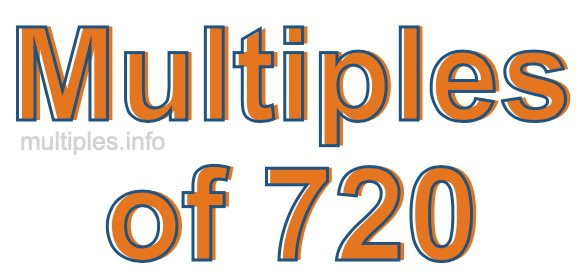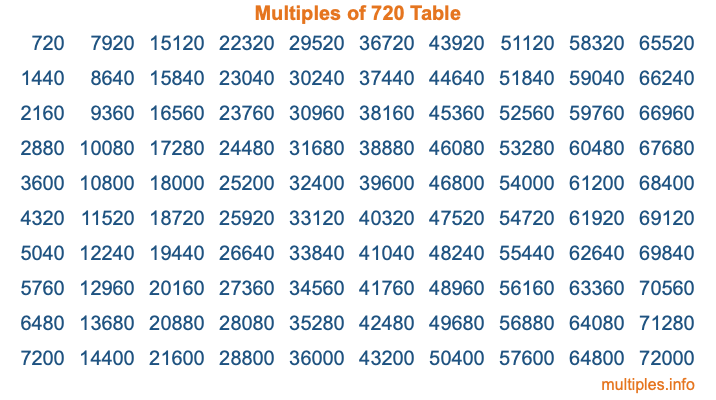Multiples of 720Welcome to the Multiples of 720 page. Here we will first teach you everything you will ever need to know about the multiples of 720, and then give you a study guide summary of everything we taught you to make sure you remember it all. Use this page to look up facts and learn information about the multiples of 720. This page will make you a multiples of seven hundred twenty expert!

Definition of Multiples of 720
Multiples of 720 are all the numbers that when divided by 720 equal an integer. Each of the multiples of 720 are called a multiple. A multiple of 720 is created by multiplying 720 by an integer.

Therefore, to create a list of multiples of 720, you start with 1 multiplied by 720, then 2 multiplied by 720, then 3 multiplied by 720, and so on for as long as you want. Thus, the list of the first five multiples of 720 is 720, 1440, 2160, 2880, and 3600. To see a larger list of multiples of 720, see the printable image of Multiples of 720 further down on this page. We also have a category where you can choose any nth multiple of 720.

Multiples of 720 Checker
The Multiples of 720 Checker below checks to see if any number of your choice is a multiple of 720. In other words, it checks to see if there is any number (integer) that when multiplied by 720 will equal your number. To do that, we divide your number by 720. If the the quotient is an integer, then your number is a multiple of 720.

Is  a multiple of 720?

Least Common Multiple of 720 and ...
A Least Common Multiple (LCM) is the lowest multiple that two or more numbers have in common. This is also called the smallest common multiple or lowest common multiple and is useful to know when you are adding our subtracting fractions. Enter one or more numbers below (720 is already entered) to find the LCM.

Check out our LCM Calculator if you need more details about the Least Common Multiple or if you need the LCM for different numbers for adding and subtraction fractions.

nth Multiple of 720
As we stated above, 720 is the first multiple of 720, 1440 is the second multiple of 720, 2160 is the third multiple of 720, and so on. Enter a number below to find the nth multiple of 720.

th multiple of 720

Multiples of 720 vs Factors of 720
720 is a multiple of 720 and a factor of 720, but that is where the similarities end. All postive multiples of 720 are 720 or greater than 720. All positive factors of 720 are 720 or less than 720.

Below is the beginning list of multiples of 720 and the factors of 720 so you can compare:

Multiples of 720: 720, 1440, 2160, 2880, 3600, etc.

Factors of 720: 1, 2, 3, 4, 5, 6, 8, 9, 10, 12, 15, 16, 18, 20, 24, 30, 36, 40, 45, 48, 60, 72, 80, 90, 120, 144, 180, 240, 360, 720

As you can see, the multiples of 720 are all the numbers that you can divide by 720 to get a whole number. The factors of 720, on the other hand, are all the whole numbers that you can multiply by another whole number to get 720.

It's also interesting to note that if a number (x) is a factor of 720, then 720 will also be a multiple of that number (x).

Multiples of 720 vs Divisors of 720
The divisors of 720 are all the integers that 720 can be divided by evenly. Below is a list of the divisors of 720.

Divisors of 720: 1, 2, 3, 4, 5, 6, 8, 9, 10, 12, 15, 16, 18, 20, 24, 30, 36, 40, 45, 48, 60, 72, 80, 90, 120, 144, 180, 240, 360, 720

The interesting thing to note here is that if you take any multiple of 720 and divide it by a divisor of 720, you will see that the quotient is an integer.

Multiples of 720 Table
Below is an image of the first 100 multiples of 720 in a table. The table is in chronological order, column by column. The first column has the first ten multiples of 720, the second column has the next ten multiples of 720, and so on.The Multiples of 720 Table is also referred to as the 720 Times Table or Times Table of 720. You are welcome to print out our table for your studies.

Negative Multiples of 720
Although not often discussed or needed in math, it is worth mentioning that you can make a list of negative multiples of 720 by multiplying 720 by -1, then by -2, then by -3, and so on, to get the following list of negative multiples of 720:

-720, -1440, -2160, -2880, -3600, etc.

Multiples of 720 Summary
Below is a summary of important Multiples of 720 facts that we have discussed on this page. To retain the knowledge on this page, we recommend that you read through the summary and explain to yourself or a study partner why they hold true.

There are an infinite number of multiples of 720.

A multiple of 720 divided by 720 will equal a whole number.

720 divided by a factor of 720 equals a divisor of 720.

The nth multiple of 720 is n times 720.

The largest factor of 720 is equal to the first positive multiple of 720.

720 is a multiple of every factor of 720.

720 is a multiple of 720.

A multiple of 720 divided by a divisor of 720 equals an integer.

720 divided by a divisor of 720 equals a factor of 720.

Any integer times 720 will equal a multiple of 720.

Multiples of a Number
Here you can get the multiples of another number, all with the same attention to detail as we did for multiples of 720 on this page.

Multiples of
Multiples of 721
Did you find our page about multiples of seven hundred twenty educational? Do you want more knowledge? Check out the multiples of the next number on our list!